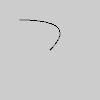Specifies a Py5Shape object’s vertex coordinates for quadratic Bezier curves.

## Examples#def setup():
py5.size(100, 100, py5.P2D)
s = py5.create_shape()
s.begin_shape()
s.no_fill()
s.vertex(20, 20)
s.end_shape()
py5.shape(s)def setup():
py5.size(100, 100, py5.P2D)
s = py5.create_shape()
s.begin_shape()
s.vertex(20, 20)
s.vertex(80, 60)
s.end_shape()
py5.shape(s)


## Description#

Specifies a Py5Shape object’s vertex coordinates for quadratic Bezier curves. Each call to quadratic_vertex() defines the position of one control point and one anchor point of a Bezier curve, adding a new segment to a line or shape. The first time quadratic_vertex() is used within a Py5Shape.begin_shape() call, it must be prefaced with a call to Py5Shape.vertex() to set the first anchor point. This method must be used between Py5Shape.begin_shape() and Py5Shape.end_shape() and only when there is no MODE parameter specified to Py5Shape.begin_shape().

Drawing 2D bezier curves requires using the P2D renderer and drawing 3D bezier curves requires using the P3D renderer. When drawing directly with Py5Shape objects, bezier curves do not work at all using the default renderer.

## Signatures#

quadratic_vertex(
cx: float,  # the x-coordinate of the control point
cy: float,  # the y-coordinate of the control point
cz: float,  # the z-coordinate of the control point
x3: float,  # the x-coordinate of the anchor point
y3: float,  # the y-coordinate of the anchor point
z3: float,  # the z-coordinate of the anchor point
/,
) -> None